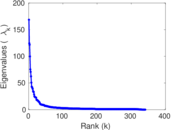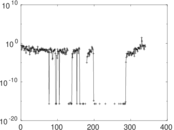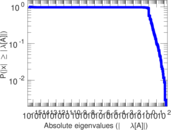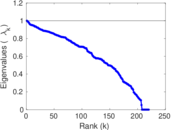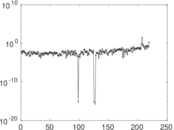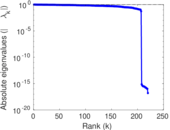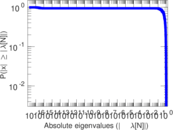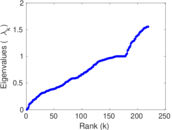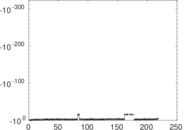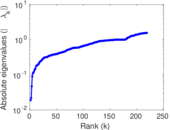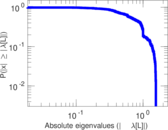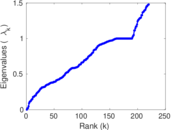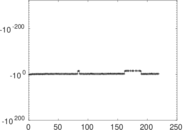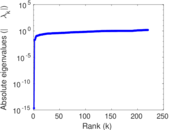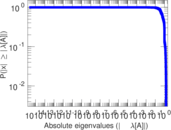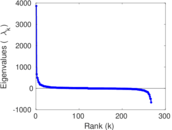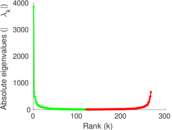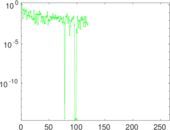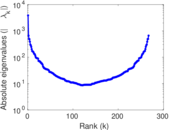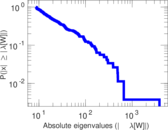# Wikibooks edits (ta)

This is the bipartite edit network of the Tamil Wikibooks. It contains users and pages from the Tamil Wikibooks, connected by edit events. Each edge represents an edit. The dataset includes the timestamp of each edit.

 Code `bta` Internal name `edit-tawikibooks` Name Wikibooks edits (ta) Data source http://dumps.wikimedia.org/ AvailabilityDataset is available for download Consistency checkDataset passed all tests Category Authorship network Dataset timestamp 2017-10-20 Node meaning User, article Edge meaning Edit Network formatBipartite, undirected Edge typeUnweighted, multiple edges Temporal dataEdges are annotated with timestamps

## Statistics

 Size n = 3,873 Left size n1 = 343 Right size n2 = 3,530 Volume m = 10,561 Unique edge count m̿ = 4,953 Wedge count s = 699,834 Claw count z = 104,364,912 Cross count x = 13,529,504,700 Square count q = 44,953 4-Tour count T4 = 3,169,878 Maximum degree dmax = 1,726 Maximum left degree d1max = 1,726 Maximum right degree d2max = 181 Average degree d = 5.453 65 Average left degree d1 = 30.790 1 Average right degree d2 = 2.991 78 Fill p = 0.004 090 72 Average edge multiplicity m̃ = 2.132 24 Size of LCC N = 3,485 Diameter δ = 14 50-Percentile effective diameter δ0.5 = 3.637 01 90-Percentile effective diameter δ0.9 = 5.785 41 Median distance δM = 4 Mean distance δm = 4.428 67 Gini coefficient G = 0.749 863 Balanced inequality ratio P = 0.202 774 Left balanced inequality ratio P1 = 0.104 725 Right balanced inequality ratio P2 = 0.280 939 Relative edge distribution entropy Her = 0.789 996 Power law exponent γ = 4.381 56 Tail power law exponent γt = 2.481 00 Tail power law exponent with p γ3 = 2.481 00 p-value p = 0.000 00 Left tail power law exponent with p γ3,1 = 1.751 00 Left p-value p1 = 0.009 000 00 Right tail power law exponent with p γ3,2 = 3.751 00 Right p-value p2 = 0.470 000 Degree assortativity ρ = −0.124 453 Degree assortativity p-value pρ = 1.486 13 × 10−18 Spectral norm α = 168.576 Algebraic connectivity a = 0.018 891 4 Spectral separation |λ1[A] / λ2[A]| = 1.355 68 Controllability C = 3,140 Relative controllability Cr = 0.826 316

## Plots

### Fruchterman–Reingold graph drawing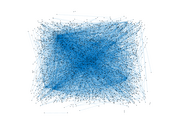### Degree distribution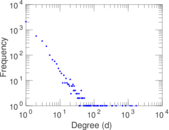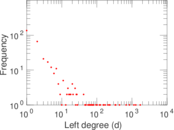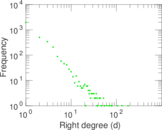### Cumulative degree distribution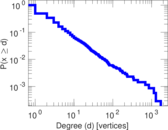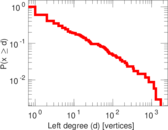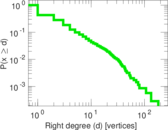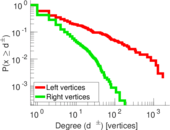### Lorenz curve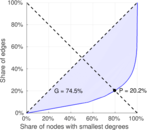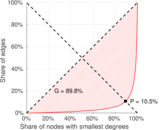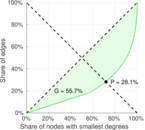### Spectral distribution of the adjacency matrix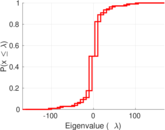### Spectral distribution of the normalized adjacency matrix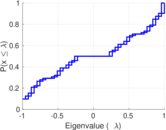### Spectral distribution of the Laplacian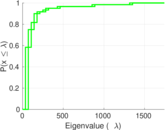### Spectral graph drawing based on the adjacency matrix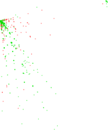### Spectral graph drawing based on the Laplacian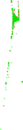### Spectral graph drawing based on the normalized adjacency matrix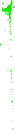### Degree assortativity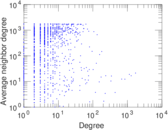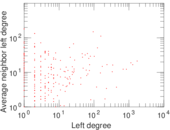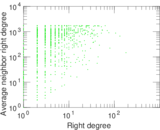### Zipf plot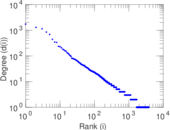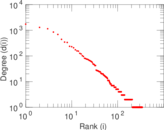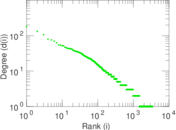### Hop distribution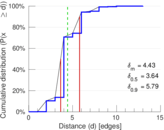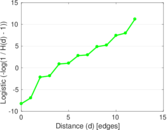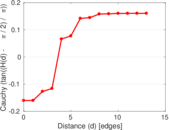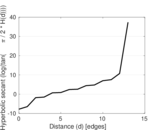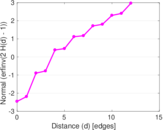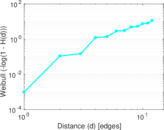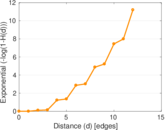### Double Laplacian graph drawing### Delaunay graph drawing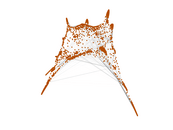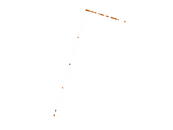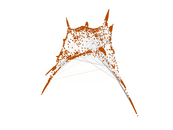### Edge weight/multiplicity distribution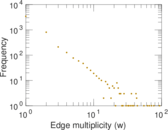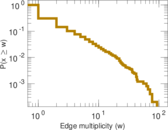### Temporal distribution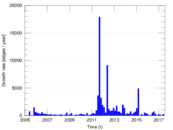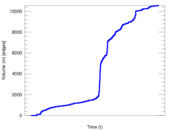### Temporal hop distribution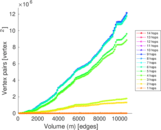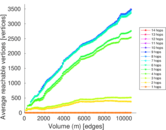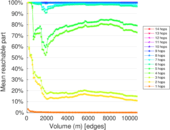### Diameter/density evolution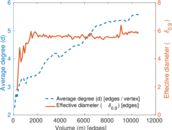### Matrix decompositions plots